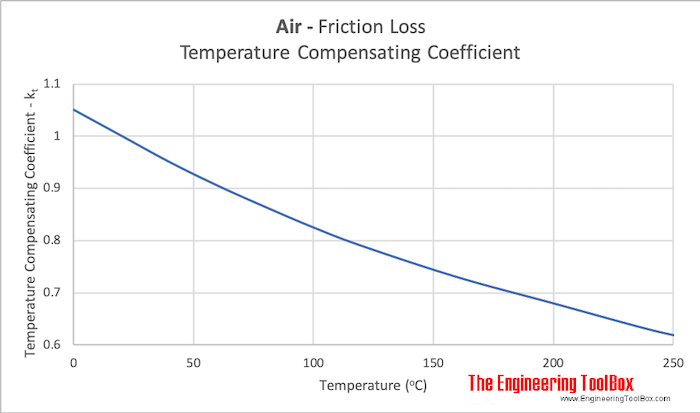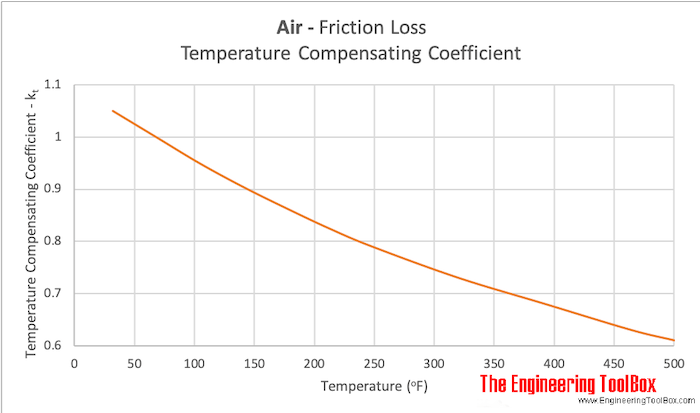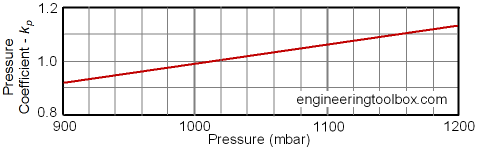Engineering ToolBox - Resources, Tools and Basic Information for Engineering and Design of Technical Applications!

# Air Ducts - Major Friction Loss vs. Temperature and Pressure

## The influence of temperature and air pressure on major friction loss.

Air ducts friction loss and pressure drop diagrams are normally made for air at Normal Temperature and Pressure (NTP) condition - with a temperature of 20 oC and a atmospheric pressure of 1 bar.

### Influence of Temperature

The friction coefficient in the D'Arcy-Weisbach Equation increases with higher temperature but the coefficient will also be outweighed by the reduced pressure loss due to reduced density of the air.

• the pressure loss in an air duct is reduced with higher temperature

With air temperature other than 20 oC - a "Temperature Compensating Coefficient" can be used to modify the pressure loss:

Δp = kt ΔpNTP         (1)

where

Δp = actual friction loss (pressure or head)

kt = Temperature Compensating Coefficient

ΔpNTP = friction loss at NTP conditions (pressure or head)

"Temperature Compensating Coefficient" for various air temperatures:### Example - Air with Temperature 100oC

For air with temperature 100oC the "Temperature Compensating Coefficient" is approximately 0.83. So,

• the pressure loss in air with temperature 100oC is approximately 83 % of the pressure loss in air at standard NTP conditions 20 oC

### Influence of Pressure

If the actual pressure differ from atmospheric pressure (1 bar abs) - friction loss in air ducts should be compensated with a "Pressure Compensating Coefficient":

Δp = kp ΔpNTP         (2)

where

Δp = actual friction loss (pressure or head)

kp = Pressure Compensating Coefficient

ΔpNTP = friction loss at NTP conditions (pressure or head)

The "Pressure Compensating Coefficient" can be estimated for various pressures from the diagram below:• 1000 mbar = 1 bar = 105 Pa (N/m2) = 0.1 N/mm2 = 10,197 kp/m2 = 10.20 m H2O = 0.9869 atm = 14.50 psi (lbf/in2) = 106 dyn/cm2 = 750 mmHg

## Related Topics

• ### Ventilation

Systems for ventilation and air handling - air change rates, ducts and pressure drops, charts and diagrams and more.

## Related Documents

• ### Air Ducts - Friction Loss Diagram

A major friction loss diagram for air ducts - SI units.
• ### Darcy-Weisbach Equation - Major Pressure and Head Loss due to Friction

The Darcy-Weisbach equation can be used to calculate the major pressure and head loss due to friction in ducts, pipes or tubes.
• ### STP - Standard Temperature and Pressure and NTP - Normal Temperature and Pressure

The definition of STP - Standard Temperature and Pressure and NTP - Normal Temperature and Pressure.

## Engineering ToolBox - SketchUp Extension - Online 3D modeling!

Add standard and customized parametric components - like flange beams, lumbers, piping, stairs and more - to your Sketchup model with the Engineering ToolBox - SketchUp Extension - enabled for use with older versions of the amazing SketchUp Make and the newer "up to date" SketchUp Pro . Add the Engineering ToolBox extension to your SketchUp Make/Pro from the Extension Warehouse !

We don't collect information from our users. More about

## Citation

• The Engineering ToolBox (2006). Air Ducts - Major Friction Loss vs. Temperature and Pressure. [online] Available at: https://www.engineeringtoolbox.com/air-ducts-temperature-pressure-friction-loss-d_1016.html [Accessed Day Month Year].

Modify the access date according your visit.

9.19.12